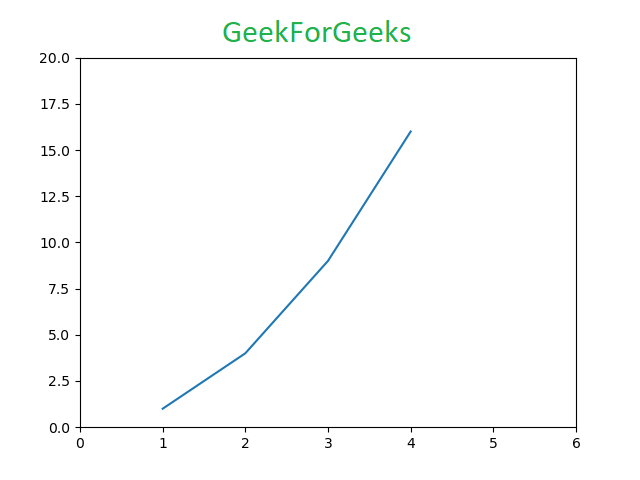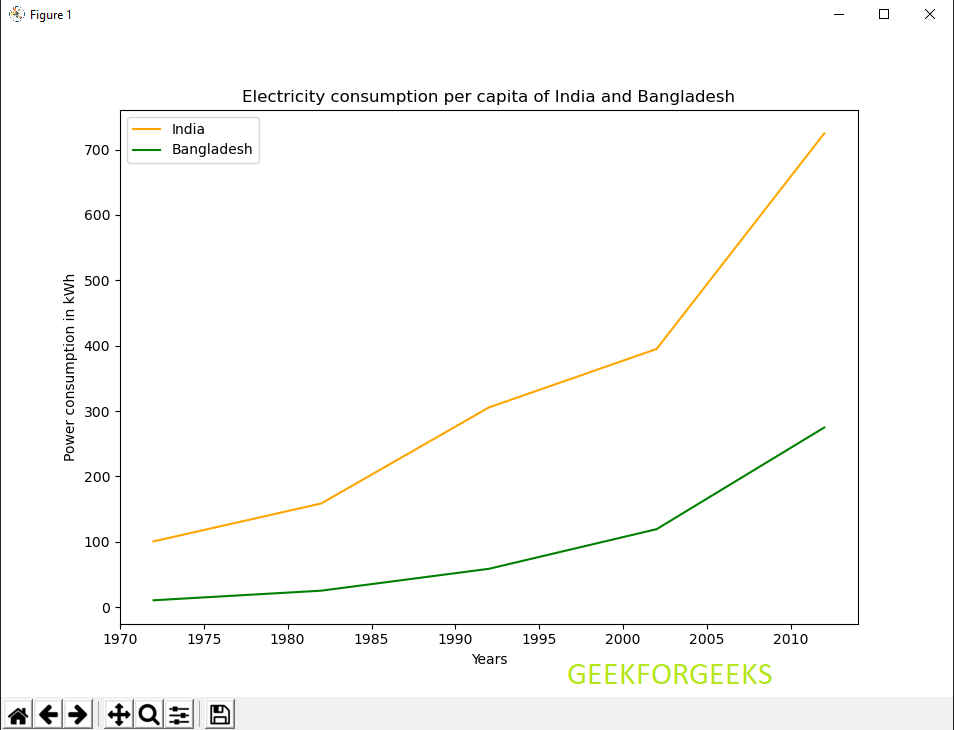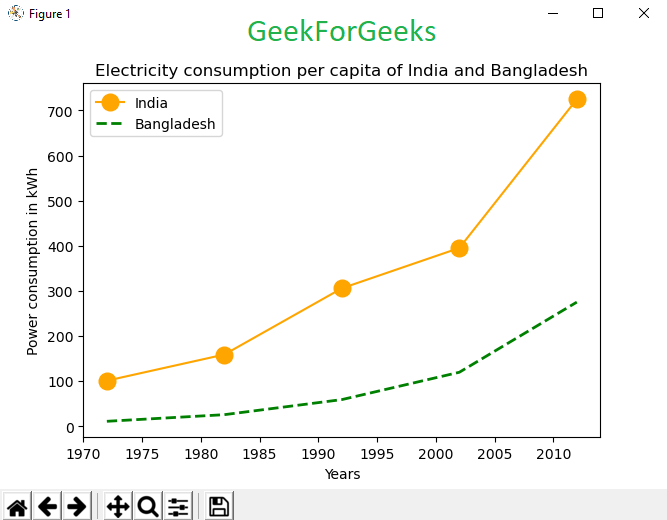Related Articles

# Pyplot in Matplotlib

• Difficulty Level : Easy
• Last Updated : 26 Mar, 2020

Matplotlib is a plotting library for creating static, animated, and interactive visualizations in Python. Matplotlib can be used in Python scripts, the Python and IPython shell, web application servers, and various graphical user interface toolkits like Tkinter, awxPython, etc.

#### Installation

To use Pyplot we must first download matplotlib module. The best way to do this is –

`pip install matplotlib`

## Pyplot

Pyplot is a Matplotlib module which provides a MATLAB-like interface. Matplotlib is designed to be as usable as MATLAB, with the ability to use Python and the advantage of being free and open-source. Each pyplot function makes some change to a figure: e.g., creates a figure, creates a plotting area in a figure, plots some lines in a plotting area, decorates the plot with labels, etc. The various plots we can utilize using Pyplot are Line Plot, Histogram, Scatter, 3D Plot, Image, Contour, and Polar.

Syntax :

matplotlib.pyplot.plot(*args, scalex=True, scaley=True, data=None, **kwargs)

To create graphs and visualizations using pyplot is quick and easy –

 `# Python program to show plot function`` ` `import` `matplotlib.pyplot as plt`` ` `plt.plot([``1``, ``2``, ``3``, ``4``], [``1``, ``4``, ``9``, ``16``])``plt.axis([``0``, ``6``, ``0``, ``20``])``plt.show()`

OUTPUT :The plot function marks the x-coordinates(1, 2, 3, 4) and y-coordinates(1, 4, 9, 16) in a linear graph with specified scales. [/caption]

Parameters: This function accepts parameters that enables us to set axes scales and format the graphs. These parameters are mentioned below :-

• plot(x, y): plot x and y using default line style and color.
• plot.axis([xmin, xmax, ymin, ymax]): scales the x-axis and y-axis from minimum to maximum values
• plot.(x, y, color=’green’, marker=’o’, linestyle=’dashed’, linewidth=2, markersize=12): x and y co-ordinates are marked using circular markers of size 12 and green color line with — style of width 2
• plot.xlabel(‘X-axis’): names x-axis
• plot.ylabel(‘Y-axis’): names y-axis
• plot(x, y, label = ‘Sample line ‘) plotted Sample Line will be displayed as a legend

For sake of example we will use Electricity Power Consumption datasets of India and Bangladesh. Here, we are using Google Public Data as a data source.

Example 1: Linear Plot

 `# Python Program to illustrate Linear Plotting`` ` `import` `matplotlib.pyplot as plt`` ` `# year contains the x-axis values``# and e-india & e-bangladesh``# are the y-axis values for plotting  `` ` `year ``=` `[``1972``, ``1982``, ``1992``, ``2002``, ``2012``]``e_india ``=` `[``100.6``, ``158.61``, ``305.54``, ``394.96``, ``724.79``]``e_bangladesh ``=` `[``10.5``, ``25.21``, ``58.65``, ``119.27``, ``274.87``]`` ` `# plotting of x-axis(year) and ``# y-axis(power consumption)``with different colored labels of two countries `` ` `plt.plot(year, e_india, color ``=``'orange'``, ``         ``label ``=``'India'``)`` ` `plt.plot(year, e_bangladesh, color ``=``'g'``, ``         ``label ``=``'Bangladesh'``)`` ` `# naming of x-axis and y-axis``plt.xlabel(``'Years'``)``plt.ylabel(``'Power consumption in kWh'``)`` ` `# naming the title of the plot``plt.title('Electricity consumption per capita\`` ``of India ``and` `Bangladesh')`` ` `plt.legend()``plt.show()`

OUTPUT :Example 2: Linear Plot with line formatting

 `# Python Program to illustrate Linear Plotting`` ` `import` `matplotlib.pyplot as plt`` ` `year ``=` `[``1972``, ``1982``, ``1992``, ``2002``, ``2012``]``e_india ``=` `[``100.6``, ``158.61``, ``305.54``, ``           ``394.96``, ``724.79``]`` ` `e_bangladesh ``=` `[``10.5``, ``25.21``, ``58.65``,``                ``119.27``, ``274.87``]`` ` `# formatting of line style and ``# plotting of co-ordinates``plt.plot(year, e_india, color ``=``'orange'``,``         ``marker ``=``'o'``, markersize ``=` `12``, ``         ``label ``=``'India'``)`` ` `plt.plot(year, e_bangladesh, color ``=``'g'``,``         ``linestyle ``=``'dashed'``, linewidth ``=` `2``,``         ``label ``=``'Bangladesh'``)`` ` ` ` `plt.xlabel(``'Years'``)``plt.ylabel(``'Power consumption in kWh'``)`` ` ` ` `plt.title('Electricity consumption per \``capita of India ``and` `Bangladesh')`` ` `plt.legend()``plt.show()`

OUTPUT :Attention geek! Strengthen your foundations with the Python Programming Foundation Course and learn the basics.

To begin with, your interview preparations Enhance your Data Structures concepts with the Python DS Course. And to begin with your Machine Learning Journey, join the Machine Learning – Basic Level Course

My Personal Notes arrow_drop_up